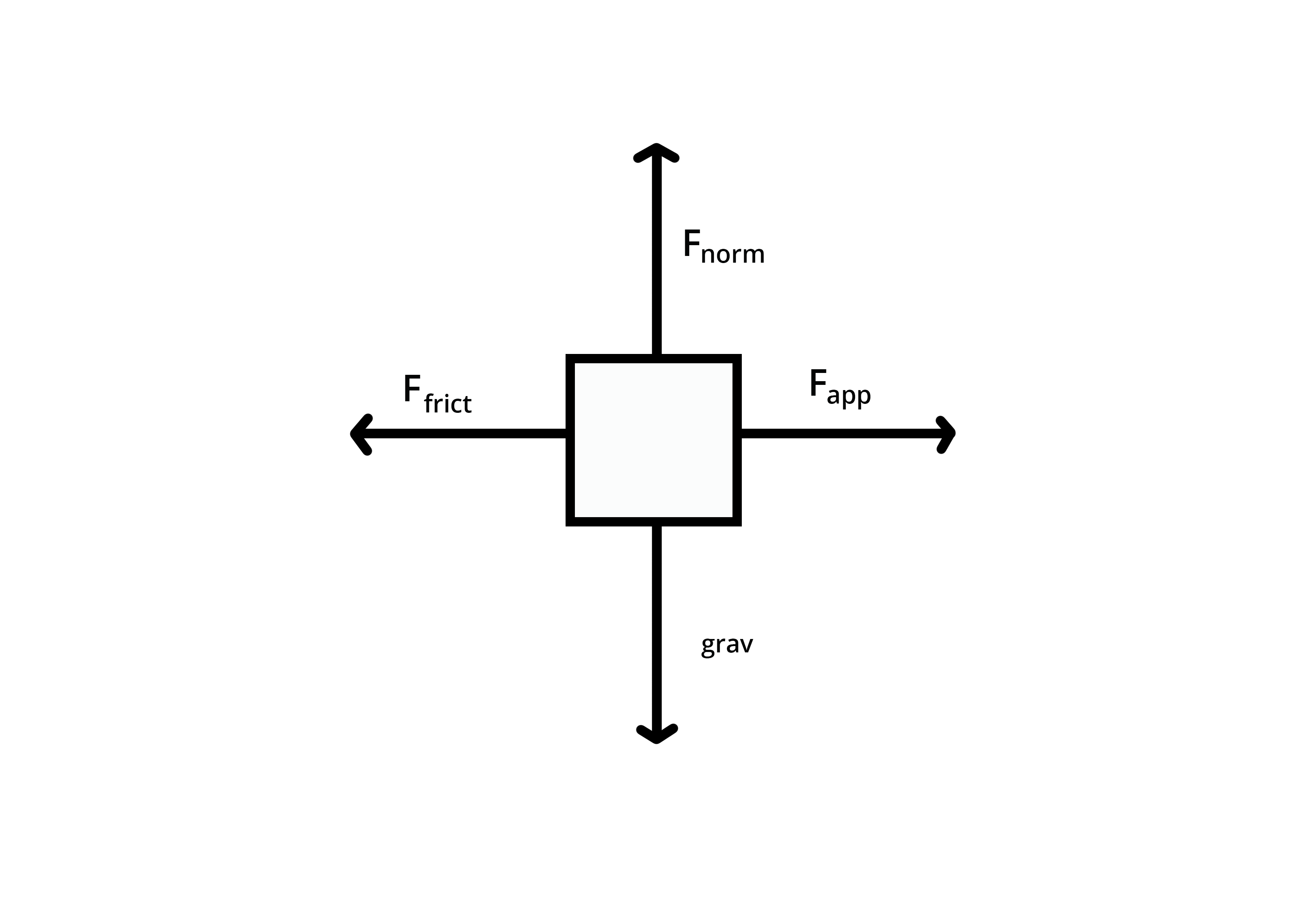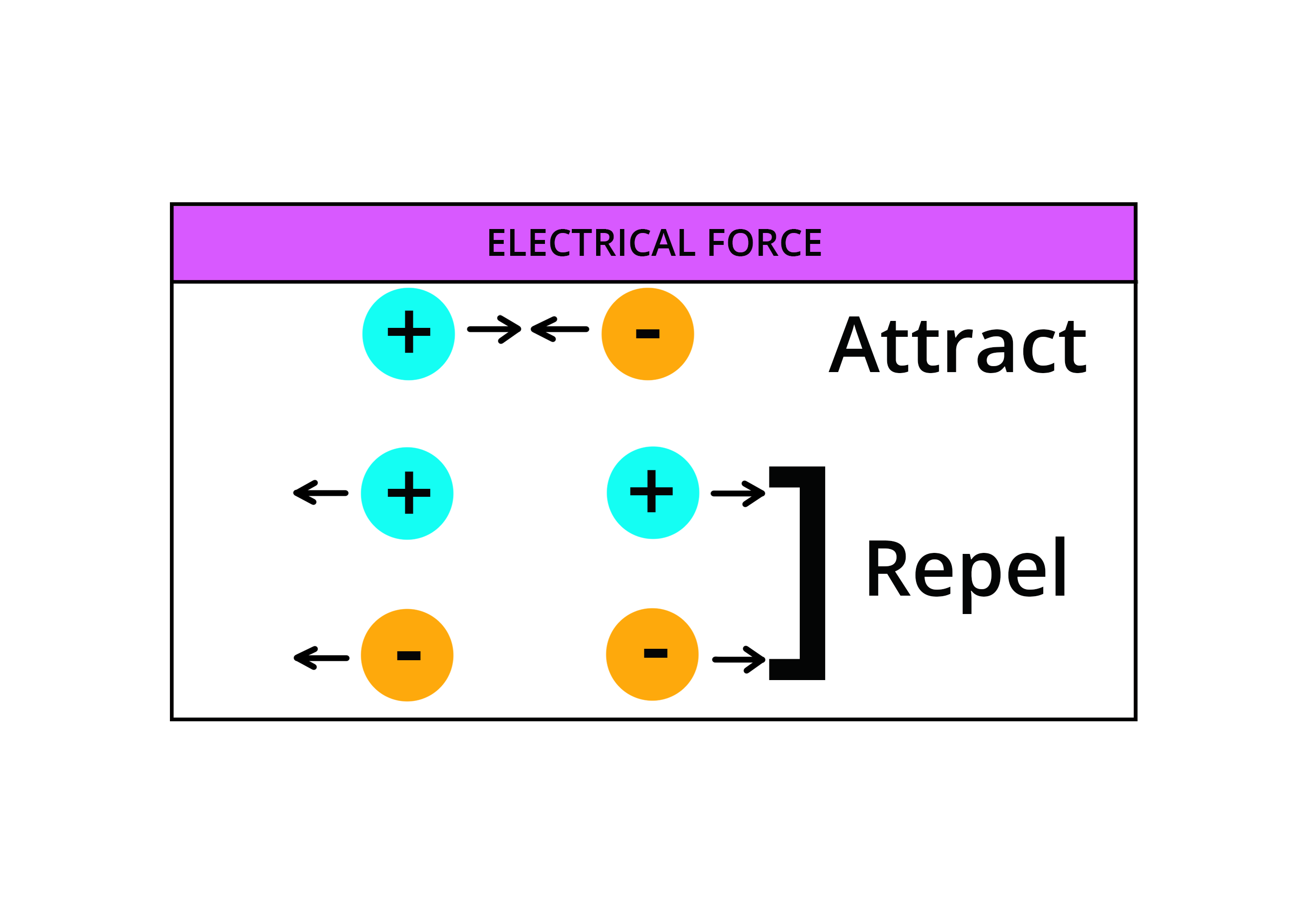• Call Now

1800-102-2727•

# Electrical Force: Electric Forces and Their Types

## Definition of electric force

An electric force is the interaction of either attractive force or repulsive force between two charged bodies. This force is similar to other forces because it affects and impacts towards a particular object and can be easily demonstrated by Newton’s law of motion. Electric force is one of the forces which is exerted over other bodies.

Furthermore, Newton’s law can be applied in order to analyze the effects of motion while this kind of force is being taken into account. To begin with, the first stage of analysis starts by constructing a free body image in which the direction of that image as well the individual force types are seen by the vector which can help calculate the total sum. This is known as the net force which is applied to a certain body to calculate its acceleration.## Size of electric force

The electric force formed among two electrons can be seen as equal to that of the electric force between two protons when locating them at equal distances. From this statement, one can clearly understand that the electric force does not depend on the mass of the body but is based on a specific quantity. This quantity is the electric charge.

## Coulomb’s law

Coulomb’s law is considered an experimental law. This law is capable of quantifying the amount of force exerted among two particles that are electrically charged. These two particles are in a stationary position. Coulomb’s force is described as the electric force between stationary charged particles. This force can also be termed electrostatic force. Coulomb’s law defines the amount of Coulomb's force or electrostatic force between stationary charges.

## Definition of Coulomb’s law

Coulomb’s law states that the amount of electrostatic force of charges that contains two points can be seen directly proportional to the scalar product of the charged particles and are indirectly (or inversely) proportional to the square of the distance between them.

## Formula of electric force

With the help of Coulomb’s law, the formula for electric force can be easily attainedWhere, F+ is the electric force which is directed among two charged particles

K is the proportionality constant

q0 is the number of charges present in one body

q1 is the number of charges contained in the other body

r is considered as the distance among the charged particles

r is the unit vector which is capable of varying

## Examples of electrical forces

Some of the common examples of electrical forces are given as follows,

• Electric circuits
• A charged bulb
• Exertion of static friction which is formed when a cloth is being rubbed by a dryer
• Feeling of shock when we suddenly hit our elbows with a doorknob

The force of electricity can be witnessed in copper wiring which is highly capable of carrying power to the entire building or industry. Electrostatic force can provide electrical energy through some of the static charges such as cathode ray tubes which are present in televisions and spray paintings which use electrostatic power sources

## Types of electric force

Electrical force consists of two different types of charges. They are positive electric charge and negative electric charge. The interaction of both of these charges is easily predictable. Unlike electric charges, they attract each other, while the like charges repel one another. This basically means that if the two charges are positive then there exists a repulsive force between both the charges.

The same thing happens if two negative charges come in contact, they both will repel each other too. In contrast, if one positive charge and one negative charge come into contact, then there is generated an attractive force, in which both the charges will be attracted towards one another. The given below is the diagrammatic representation of the same,## Electric force and charged particles

Electric force can happen among all the charged particles irrespective of the type of charge. These are seen as tiny particles and can be found inside atoms. They are termed protons and electrons. Protons are made up of positively charged particles whereas electrons are built by negatively charged ones. The other objects which are produced from atoms become charged due to an imbalance of the number of electrons and protons contained inside the atoms.

In an atom, protons are present inside the nucleus and are very tightly bound. They cannot move or travel across the atoms or nucleus. Meanwhile, electrons can be seen away from the atoms in their own orbitals. They can move around the atom.Talk to our expert
Resend OTP Timer =
By submitting up, I agree to receive all the Whatsapp communication on my registered number and Aakash terms and conditions and privacy policy